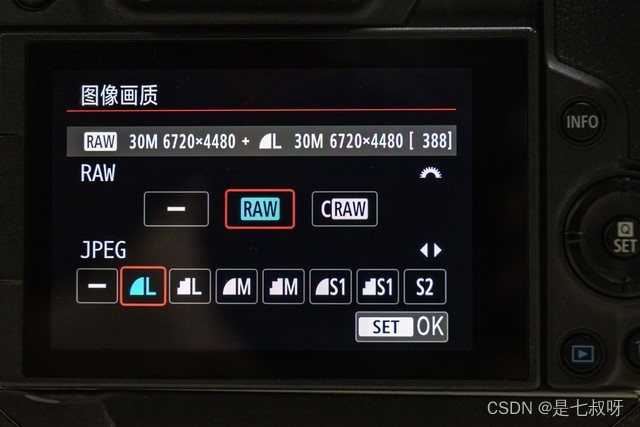# 一、RAW图像详解

## 1.1 什么是raw格式图像？

RAW在英文中的解释是未处理的、自然状态的，这也就是RAW文件的真谛。RAW图像就是CMOS或者CCD图像感应器将捕捉到

## 1.2 RAW格式与JPG格式有什么差别？## 1.3 那么RAW和JPG格式到底有什么比较大的区别呢？

`RAW我们通常用色彩深度来表示，色彩深度的单位是bit，一般的JPG照片色彩深度为8bit`，这意味着RGB这三种颜色，每个颜色有256个等级，组合起来可以产生总共256256256即约1677万种不同的颜色。

# 二、使用Python读取raw格式图像并显示

• 一律使用`np.fromfile`读取之后再转化为`.png`或者`.tff`格式，`读取的时候直接传入文件路径，不用加'r'`
• ## 2.1 输出为png图像显示

``````import os.path
import os
from PIL import Image
import numpy as np
import cv2
import imageio

def read(input_dir, shape, save_dir):
if not os.path.exists(save_dir):
os.makedirs(save_dir)
# img = cv2.imread('D:\Installer\\h_1_3_00_2_4_0_0_5_30_01_0_00.raw')

# type = img.dtype
w, h, c = shape
input_dir = os.path.join('D:\\JS17\rawData\\', input_dir)
list = os.listdir(input_dir)
for path in list:
if path.endswith('.xml'):
continue
### 直接传入文件路径，不用加'r'
imgData = np.fromfile(input_dir + '\\' + path, dtype='uint16')   ### 这里是16bit所以要uint16
w, h, c = 5120, 4096, 1
# # imgData = np.fromfile(r'D:\Installer\\h_1_1_0_2_0_4_1_5_38_63_0.raw', dtype='uint16')   # SAR
# # w, h, c = 2048, 2048, 1
imgData = imgData.reshape(w, h, c)
cv2.imwrite(save_dir + path.split('.') + '.png', imgData)
if __name__ == '__main__':
#### 传入raw文件夹；图像的w, h, c；图像的保存路径
read('SAR', [2048, 2048, 1], 'D:\\JS17\TIFData\SAR\\')
read('中波红外', [1024, 1280, 1], 'D:\\JS17\TIFData\MiddleHW\\')
read('可见光', [4096,5120, 1], 'D:\\JS17\TIFData\KJG\\')
``````

## 2.2 输出为tif图像显示

``````import imageio
import numpy as np

raw=np.memmap('140-200-1000（2）.raw',dtype=np.uint16,shape=(4288,3524))#读取16位raw图片
imageio.imsave('raw.tif',raw)#转换格式与保存

### 上面两行其实就已经读取并且转换完成了，已经可以显示了
### 下面是做裁剪的，仅供参考
tif=np.memmap('raw.tif',dtype=np.uint16,shape=(4288,3524))#读取16位tif图片
resize=tif[1200:1700,1200:1700]
imageio.imsave('resize.tif',resize)#对16位tif图片裁切
``````